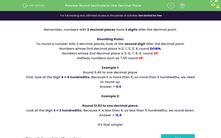# Practise Rounding Decimals to One Decimal Place

In this worksheet, students will be asked to round decimal numbers with two decimal places, to one decimal place.Key stage:  KS 2

Curriculum topic:   Number: Fractions, Decimals and Percentages

Curriculum subtopic:   Round Up/Down Decimals

Popular topics:   Rounding worksheets, Estimation worksheets

Difficulty level:#### Worksheet Overview

In this activity, we will be practising rounding decimal numbers.

Remember, numbers with 2 decimal places have 2 digits after the decimal point.

Rounding Rules:

To round a number with 2 decimal places, look at the second digit after the decimal point.

Numbers whose 2nd decimal place is 0, 1, 2, 3, 4, round DOWN.

Numbers whose 2nd decimal place is 5, 6, 7, 8, 9, round UP.

Halfway numbers such as 7.55 round UP.

Let's try some example questions.

Example 1

Round 6.46 to one decimal place.

First, look at the digit 6 in the secoed decimal place - it means 6 hundredths. Because it is more than 5 hundredths, we need to round up.

6.46 rounds up to 6.5.

Example 2

Round 12.83 to one decimal place.

Look at the digit 3 which means 3 hundredths. Because it is less than 5 hundredths, we round down.

12.83 rounds down to 12.8.

It's that simple!

Now you can try some questions like this.### What is EdPlace?

We're your National Curriculum aligned online education content provider helping each child succeed in English, maths and science from year 1 to GCSE. With an EdPlace account you’ll be able to track and measure progress, helping each child achieve their best. We build confidence and attainment by personalising each child’s learning at a level that suits them.

Get started#### Popular Maths topics

••••